Function Repository Resource:

Find a quadric surface that passes through nine given 3D points

Contributed by: Ed Pegg Jr
 ResourceFunction["NinePointQuadric"][pts,{x,y,z}] returns the implicit Cartesian equation in the variables x,y and z of the quadric surface that goes through the points pts. ResourceFunction["NinePointQuadric"][pts] uses the formal variables x, y and z.

## Examples

### Basic Examples (3)

Find the quadric surface going through nine points (based of the decimal digits of 927):

 In:=Out=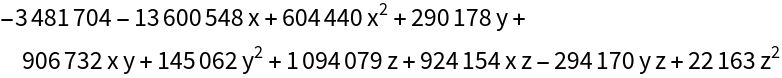Show the quadric surface (a hyperboloid of one sheet) with the points:

 In:=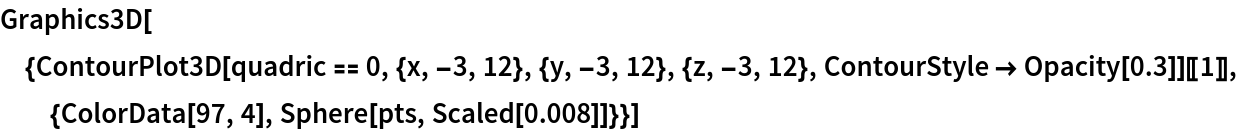Out=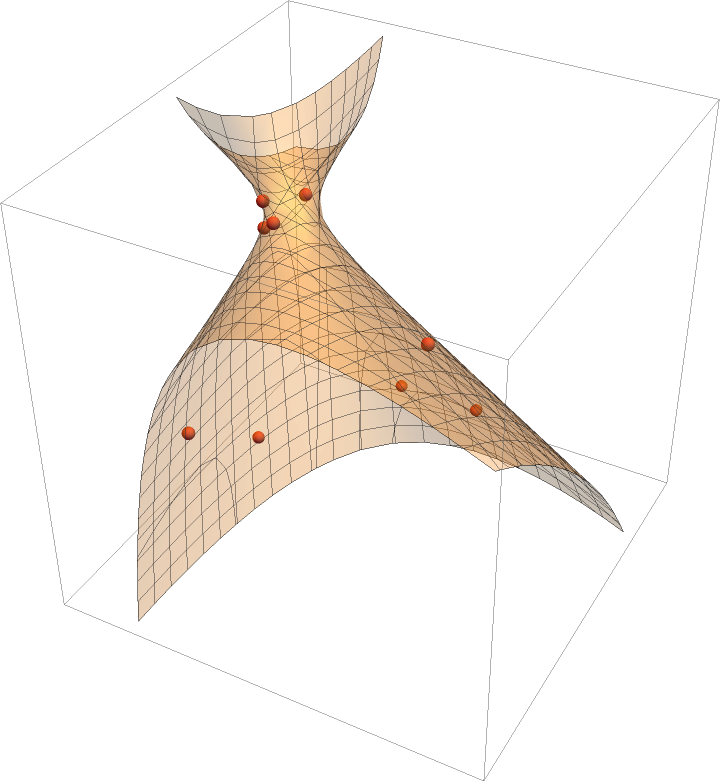Find the quadric surface going through nine points (based of the decimal digits of 928):

 In:=Out=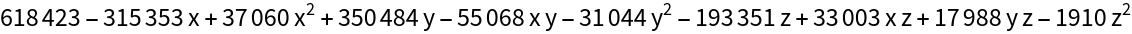Show the quadric surface (a hyperbolic paraboloid) with the points:

 In:=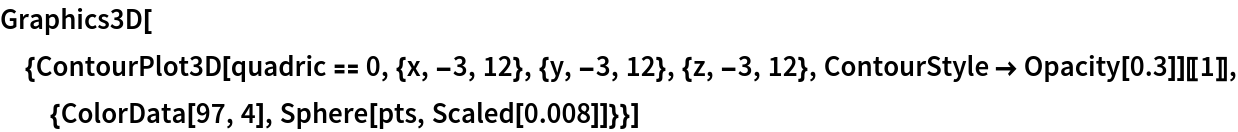Out=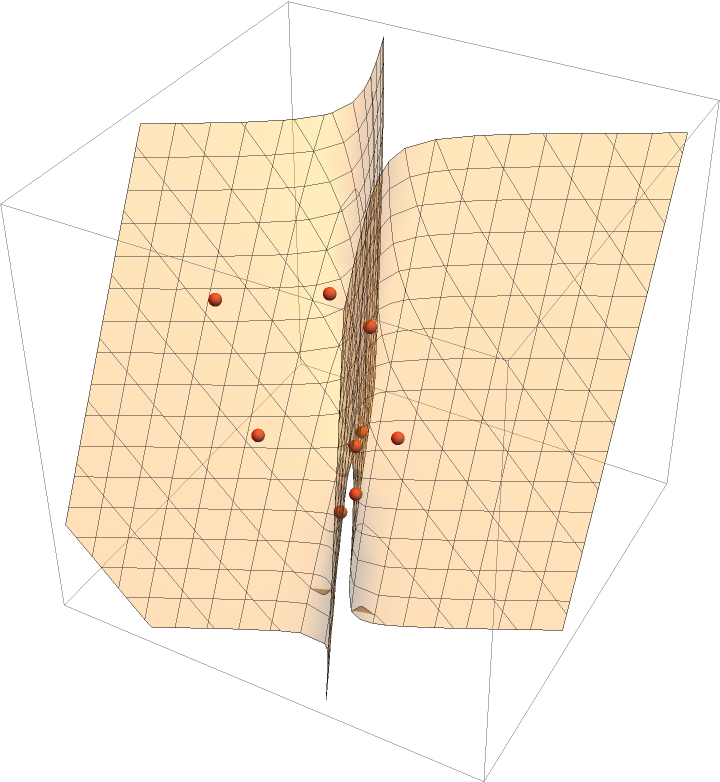Find the quadric surface going through nine points based of the decimal digits of 925:

 In:=Out=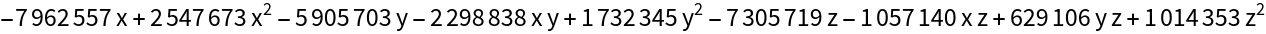Show the quadric surface (an ellipsoid) with the points:

 In:=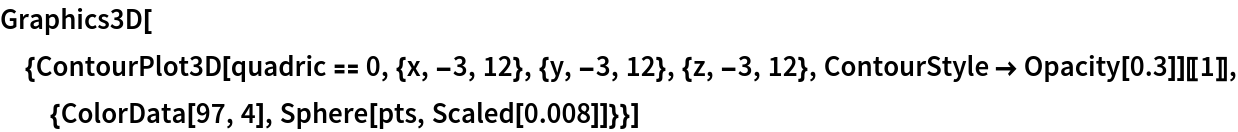Out=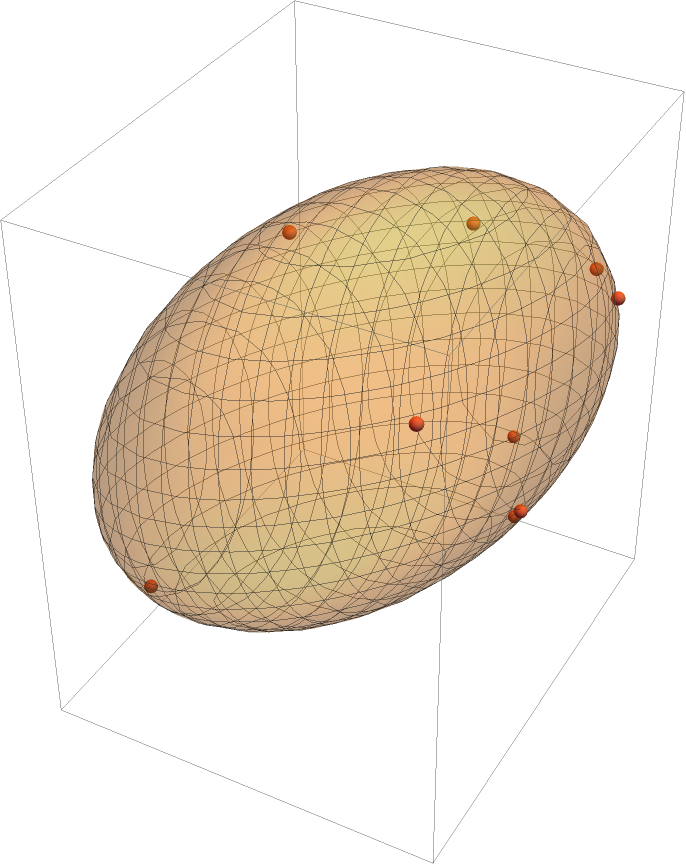Use the resource function QuadricSurfacePlot to show this same ellipsoid:

 In:=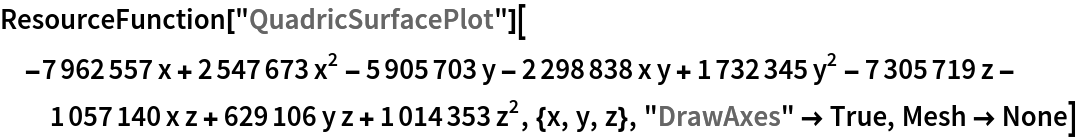Out=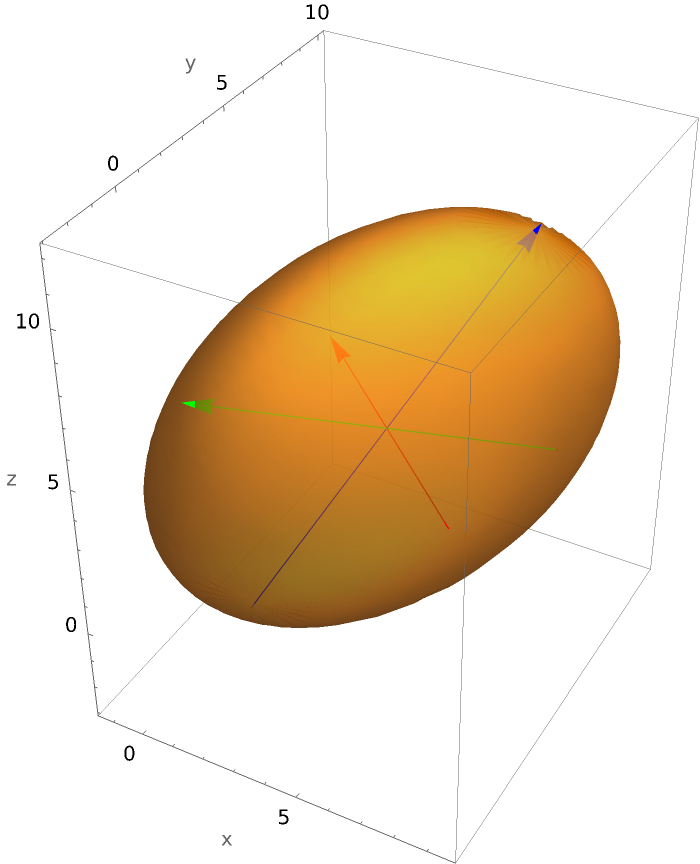### Scope (2)

Use formal variables:

 In:=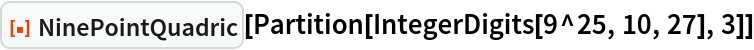Out=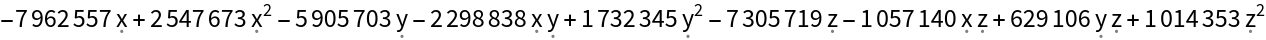Nine random real points:

 In:=Out=In:=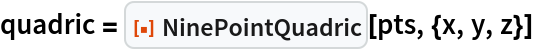Out=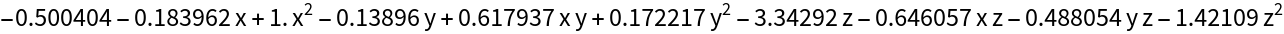Show the quadric surface (a hyperboloid of 2 sheets) with the points:

 In:=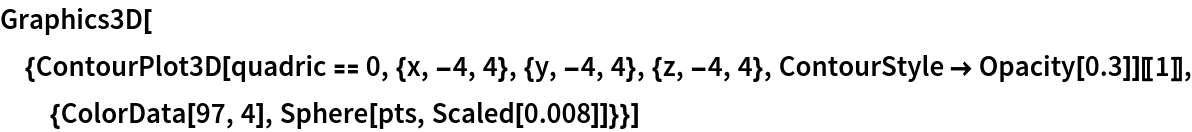Out=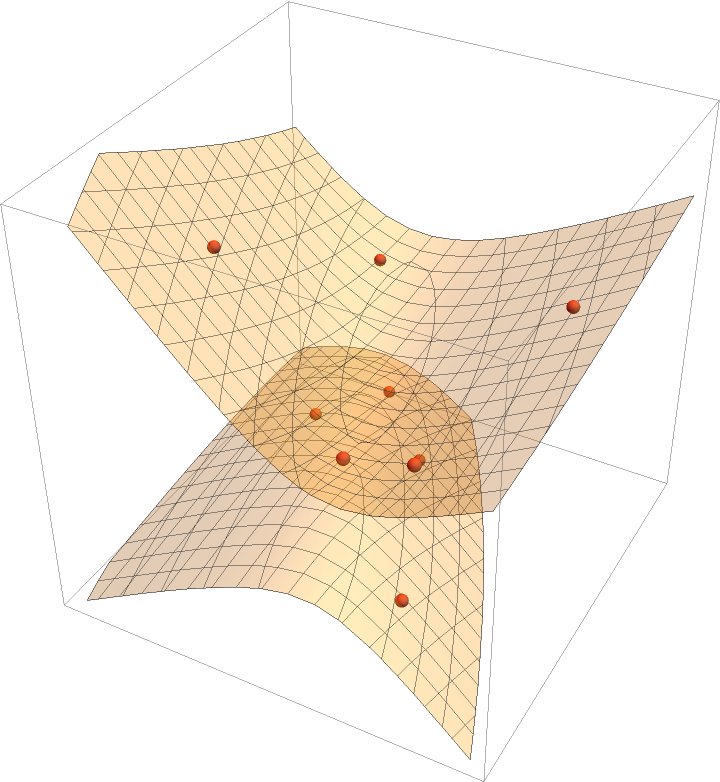### Possible Issues (3)

Pick nine points on a sphere:

 In:=Out=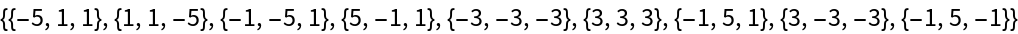Find the surface of the sphere:

 In:=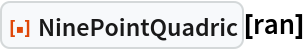Out=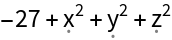The following set of nine points should give the same sphere equation, but the matrix method fails due to degeneracy:

 In:=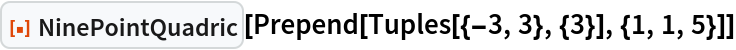Out=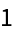## Version History

• 1.0.0 – 25 July 2022